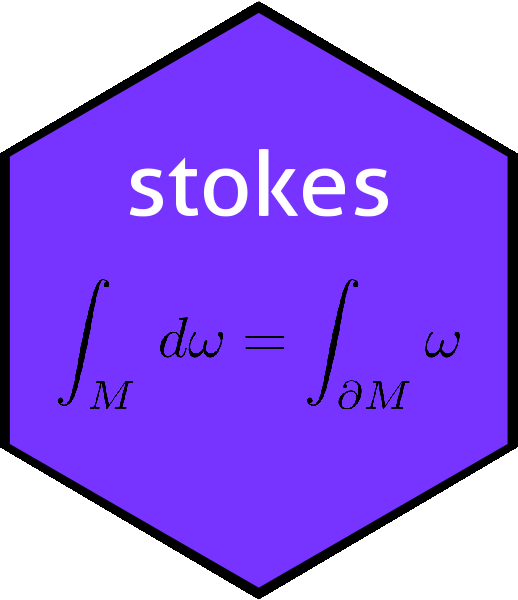# Objects ex, ey, and ez in the stokes packageex <- e(1,3)
ey <- e(2,3)
ez <- e(3,3)

Convenience objects ex, ey, and ez are discussed here. Elementary forms dx, dy and dz are discussed in dx.Rmd.

The dual basis to $$(dx,dy,dz)$$ is, depending on context, written $$(e_x,e_y,e_z)$$, or $$(i,j,k)$$ or sometimes $$\left(\frac{\partial}{\partial x},\frac{\partial}{\partial x},\frac{\partial}{\partial x}\right)$$. Here they are denoted ex, ey, and ez (rather than i,j,k which cause problems in the context of R).

fdx <- as.function(dx)
fdy <- as.function(dy)
fdz <- as.function(dz)
matrix(c(
fdx(ex),fdx(ey),fdx(ez),
fdy(ex),fdy(ey),fdy(ez),
fdz(ex),fdz(ey),fdz(ez)
),3,3)
##      [,1] [,2] [,3]
## [1,]    1    0    0
## [2,]    0    1    0
## [3,]    0    0    1

Above we see that the matrix $$dx^i\frac{\partial}{\partial x^j}$$ is the identity, showing that ex, ey, ez are indeed conjugate to $$dx,dy,dz$$.

### Package dataset

Following lines create exeyez.rda, residing in the data/ directory of the package.

save(ex,ey,ez,file="exeyez.rda")